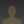# Fooling Yourself with Quantum Mechanicschipveres wrote 12/13/2017 at 14:13 • 1 min read • Like

Here's how to deceive yourself in three easy steps:

1.  Use Newton's gravity equation to calculate the position of a planet.  It works fine, but in its most common form contains the assumption that all the mass of the planet is concentrated at a single point in the center.

2.  Use Schrodinger's equation to calculate the position of an electron.  Still works fine, still contains the same assumption.

3.  Conclude from step two that the electron is a point particle.  Don't notice that the assumption is also the conclusion.

## Discussions